# Why Do We Use Decision Trees in Machine Learning?Decision trees in machine learning (ML) are used to structure algorithms. A decision tree algorithm helps split dataset features with a cost function. Through a process called pruning, the trees are grown before being optimized to remove branches that use irrelevant features. Parameters like decision tree depth can be set to lower the risk of an overly complex tree or overfitting.

Decision trees are used to solve classification problems and categorize objects depending on their learning features. They can also be used for regression problems or as a method to predict continuous outcomes from unforeseen data.

This article will look at the need to use decision trees in ML, the different types of decision trees, the pros and cons, and much more.

## What is a decision tree?

A decision tree is a way of modeling outcomes and decisions with each decision in the branching structure representing a different branch. It is used to calculate the success potential of various resolutions to achieve a specific goal.

Being manual models for portraying operational decisions, decision trees existed long before the invention of machine learning. They continue to be used by businesses to analyze organizational decisions.

A form of predictive modeling, decision trees help map various solutions or decisions that arise for a given outline. Different nodes are required to make the trees. The beginning of the tree is the root node, which is a whole dataset that lies within machine learning.

The leaf node is the final output of a series of decisions made or the endpoint of a branch. There will not be more after the leaf node. With regard to machine learning, the data features are the internal nodes and the outcomes are the leaf nodes.

Decision trees can be used with a supervised machine learning model. The technique uses labeled input and datasets for output to train a model. This approach can be employed to solve classification issues when an object needs to be classified or categorized.

Decision trees can be applied to regression issues as an approach in predictive analytics to forecast outputs from unseen data. They are popular in the machine learning community as forms of structured models. The tree-like structure is easy to understand and allows us to analyze the decision-making process quickly.

Explainability, which refers to understanding a model’s output, is a vital part of machine learning. It is a powerful tool that detects flaws in the model and influences the data. It helps verify predictions to improve model performance and gains new insights about a problem.

## Understanding the decision tree algorithm

The decision tree algorithm belongs to the supervised learning algorithm family. The main goal is to create a training model to predict the value of the target variable by learning simple calculations from the previous data.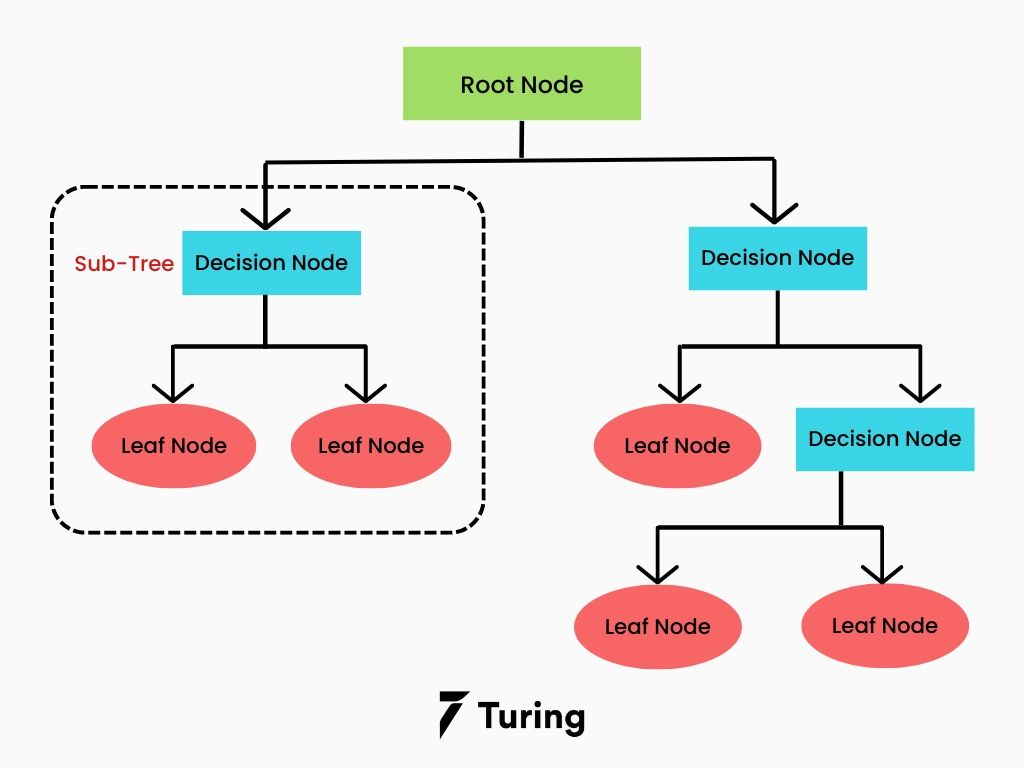To predict a class label for a record in decision trees, we need to start from its root. We have to compare the values of the root attribute with the record’s attribute. We then follow the branch that suits the value and jump to the next one. We use the pruning technique to generate more branches in a tree.

## Decision tree terminologies

Here are some common terminologies related to decision trees.

• Root node: Represents the total population or a tiny portion of it. Root nodes can be divided into two or more homogeneous datasets.
• Decision node: A sub-node that is further divided into sub-nodes.
• Pruning: The process of deleting a sub-node from a decision node.
• Splitting: A process to divide a node into different subnodes.
• Terminal or leaf node: The nodes that don’t split.
• Parent and child node: A node divided into sub-nodes. The sub-nodes from the parent node are known as the child node.
• Branch or sub-tree: A subset of the entire tree.

Using the classification example, we can sort a decision tree from the root to the terminal nodes. Every node will act as a test case and every edge will descend from the node to the corresponding possible solutions for the test case. We repeat this recursive process until every subtree is rooted in the new role.

## Types of decision trees in machine learning

Depending on the target variable, decision trees can be divided into two types: continuous variable and categorical variable decision trees.

### Continuous variable decision tree

A continuous variable decision tree is one that has an uninterrupted target variable. Example: a person can predict what his income is likely to be by using the information that is already available, such as his age, occupation, and other continuous variables.

### Categorical variable decision tree

A categorical variable decision tree has an unconditional target variable divided into various types. For instance, the categories can be either yes or no. The types mean that each stage of the decision-making process falls into each category and there is nothing in-between.

## How do decision trees work?

We need to know a tree’s accuracy to arrive at a decision to build strategic splits. The decision criteria varies based on regression and classification trees. Decision trees use different algorithms to split a node into different sub-nodes. Building sub-nodes increases the homogeneity of the forthcoming sub-nodes.

The purity of the node increases depending on the target variable. The decision tree will split the nodes on all variables and then choose the split that results in the most homogenous sub-nodes. The algorithm selection largely depends on the target variable type.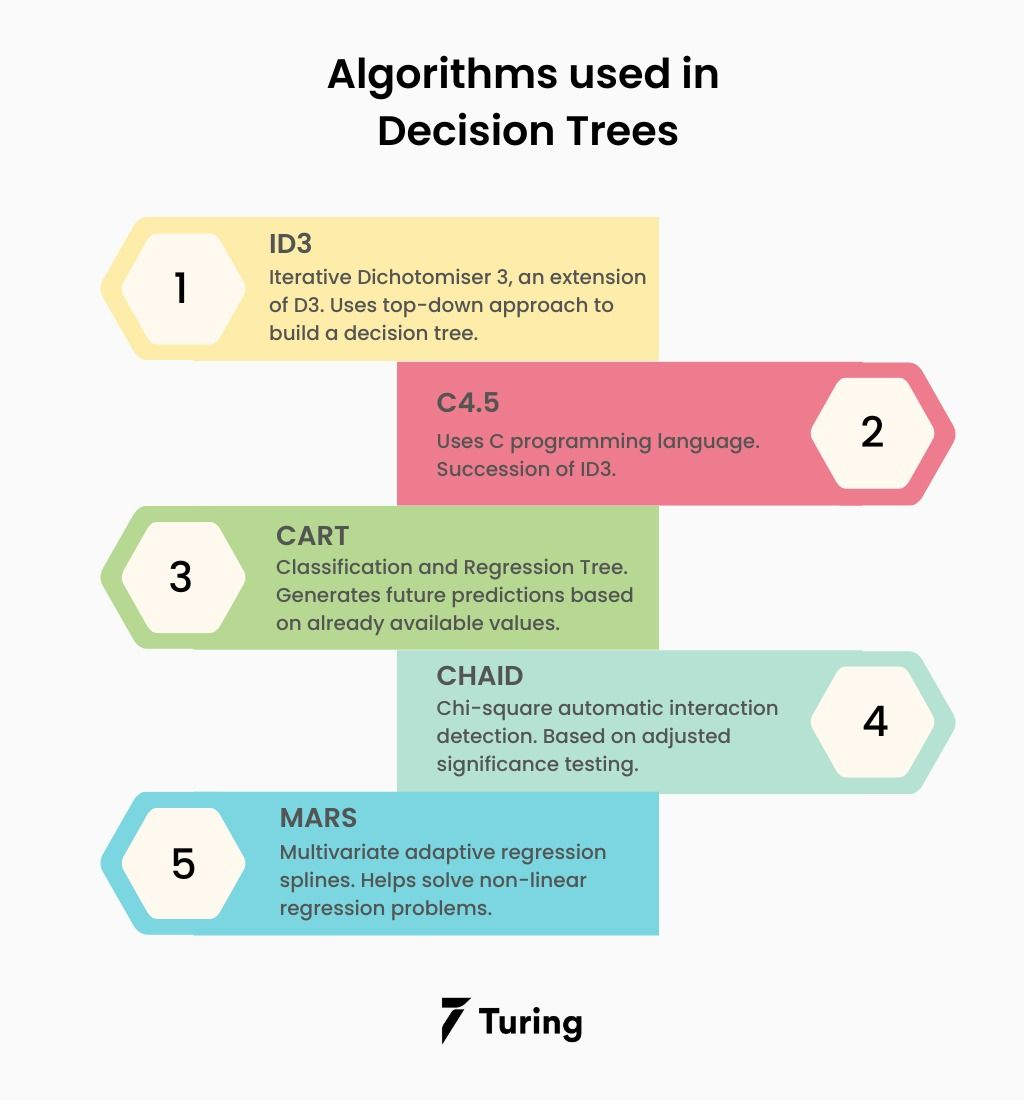The following are the algorithms used in decision trees.

### ID3

ID3 or Iterative Dichotomiser 3 is an algorithm used to build a decision tree by employing a top-down approach. The tree is built from the top and each iteration with the best feature helps create a node.

Here are the steps:

• The root node is the start point known as a set S.
• Each iteration of the algorithm will iterate through unused attributes of the root node and calculate the information gain (IG) and entropy (S).
• It will select the attribute with the tiniest entropy or higher information gain.
• We divide set S by choosing the attribute to produce the data subset.
• The algorithm will continue if there is no repetition in the attributes chosen.

### C4.5

The C4.5 algorithm is an improved version of ID3. C in the algorithm indicates that it uses C programming language and 4.5 is the algorithm’s version. It is one of the more popular algorithms for data mining. It is also used as a decision tree classifier and to generate a decision tree.

### CART

Classification and Regression Tree or CART is a predictive algorithm used to generate future predictions based on already available values. These algorithms serve as a base of machine learning algorithms like bagged decision trees, boosted decision trees, or random forests.

There are marked differences between regression trees and classification trees.

• Regression trees: Predict continuous values depending on information sources or previous data. For instance, to predict the price of an item, previous data needs to be analyzed.
• Classification trees: Determine whether an event occurred. It usually has outcomes as either yes or no. This type of decision tree algorithm is often used in real-world decision-making.

### CHAID

Chi-square automatic interaction detection (CHAID) is a tree classification method that finds the importance between the parent nodes and root nodes. It is measured by adding the squares of standardized differences between the expected and observed frequencies of the target variable.

It works using the categorical target variables, Success or Failure, and can work on two or more splits. If the Chi-square value is high, the statistical importance of the variation of the parent node and root nodes will also be high. It will generate CHAID.

### MARS

Multivariate adaptive regression splines or MARS is a complex algorithm that helps solve non-linear regression problems. It lets us find a set of linear functions that provide the best prediction. It is a combination of simple linear regression functions.

## Attribute selection measures

If a dataset contains N attributes, choosing what attribute to place at the root node or different tree levels as internal nodes can be complex. Randomly selecting a node as the root won’t solve the problem either. Following a random approach will also produce bad results with very low accuracy. To address this attribute-choosing problem, we need to apply the solutions below:

• Entropy
• Information gain
• Gini index
• Gain ratio
• Reduction in variance

These solutions help calculate the values for each attribute. We can sort the values and place them in the tree with the higher value to the root and lower as it goes to the sub-nodes. Note that if we use information gain, we need to consider attributes as categorical. On the other hand, if we use Gini index, we should consider them as continuous.

### Entropy

Entropy is a measure of the randomness of the information being processed. The higher the entropy, the harder it will be to solve that information. For example, when we flip a coin, we can’t be sure about the outcome. We are simply performing a random act that will provide a random result.

In ID3, a branch with zero entropy is denoted as a leaf node. The one with entropy more than zero will need splitting.

The formula for the entropy of one attribute is:where Pi is the probability of an event i from the S state.

### Information gain

Information gain is a statistical characteristic that measures how well an attribute divides the training instances according to their target types. Building a decision tree is discovering attributes that will return the best information gain and the smallest entropy.

Information gain is a decline in entropy. It computes the difference between entropy before the dataset split and the average entropy after the dataset split depending on the specified attribute values.

The formula is as below:where before is the dataset previous to the split, (j, after) is the subset j next to the split, and K is the subset quantity created by the split.

### Gini index

The Gini index is a measure of purity or impurity that is used when creating a decision tree in the CART algorithm. Comparison of the attribute with a lower Gini index is possible only with an attribute with a higher Gini index. The index can create only binary splits, and the CART algorithm uses it to create the same.

We can use a cost function for evaluating splits in the dataset denoted using the Gini Index. We can calculate it by subtracting the total of the squared probabilities of every class from one. It will favor huge partitions and is very easy to implement, but IG will gain fewer partitions with unique values. The Gini Index formula is as below: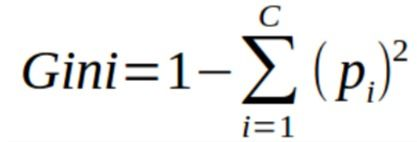### Gain ratio

Information gain is influenced by selecting attributes with higher values as root nodes. It favors the attributes with higher and unique values. C4.5 is an advancement of ID3, and the gain ratio is a modification of information gain that reduces the influence to make it the best option.

The gain ratio conquers the issue of using information gain by considering the branch count which would result before the split. It corrects information gain by getting the inherent information of a split.

The formula for the gain ratio is as follows: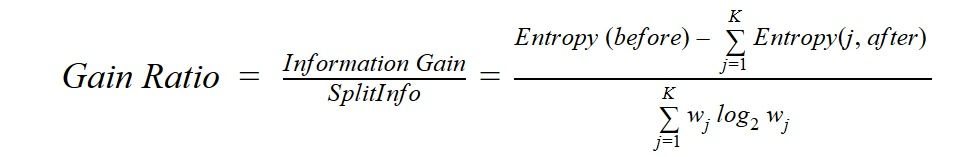where before is the dataset before the split. (j, after)is the subset next to the split and K is the subset quantity generated because of the split.

### Reduction in variance

Reduction in variance is an algorithm for regression issues or continuous target variables. It uses the usual formula of variance to select the perfect split. A lower variance split is the criteria for the population split. The formula is: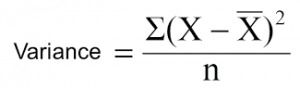where X-bar is the mean of the values, n is the total values, and X is actual.

## How to avoid or counter overfitting in decision trees

The major problem with a decision tree is that it has a table full of attributes to the extent that fitting more is difficult. It can be hard to train a dataset when there is so much information. If there is no set limit for the tree, we can achieve 100% training accuracy. In the worst case, it will create one leaf per observation which will impact the accuracy when we want to predict samples that aren’t in the training set.

Here are two ways to remove overfitting: pruning and random forest.

### Pruning

The splitting process will provide a grown tree until it reaches the final stage. However, the fully grown tree overfits the data which leads to poor accuracy on unseen data. In pruning, the decision nodes are cut off from the leaf node.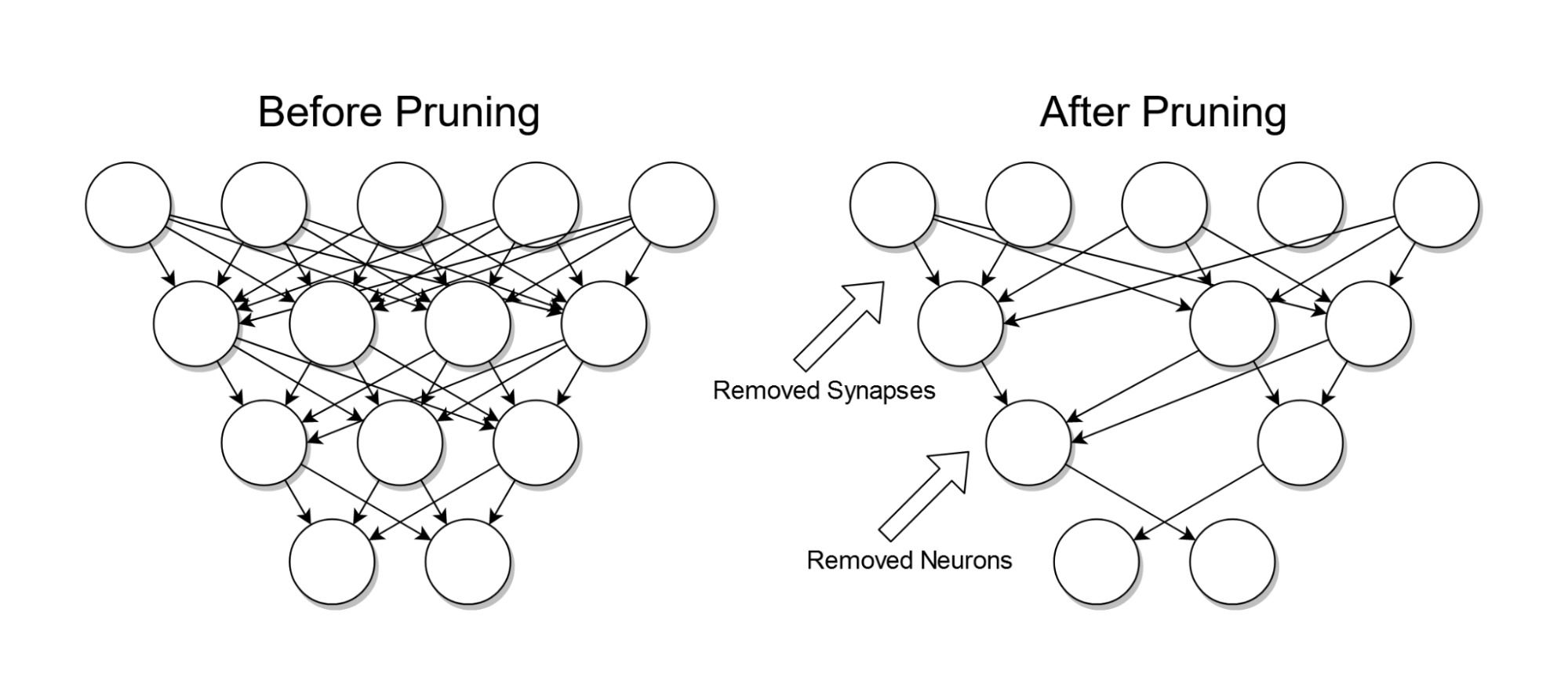Image source: Wikipedia

The training set can be filtered into two sets: the validation dataset and the training dataset. The decision tree can be built by filtering the training dataset. The trimming process can continue until the accuracy needed is achieved.

### Random forest

Random forest is an example of ensemble learning where different machine learning algorithms are joined to obtain predictive performance. There are two reasons it is called random: one, because we create a random training dataset when creating trees, and two, because we use the random feature subsets when splitting the nodes. We use the bagging technique to create an ensemble of trees to create different training sets with replacements.

## Linear model vs. tree-based model

The decision to choose between a linear and tree-based model depends on the issues we want to resolve. Below are a few scenarios:

• A tree-based model will outperform the classic regression approach if there is higher non-linearity and a complex relationship with independent and dependent variables.
• Linear regression will outperform the tree-based approach if the relationship between dependent and independent variables is measured using a linear model.

When building a model, a decision tree approach is a better choice as it is simpler to interpret compared to a linear regression model.

The advantages of decision trees are as follows.

• Easily understandable: Decision trees are easy to understand and interpret as they provide a way to visualize algorithms.
• Low or no requirement for data preprocessing: Unlike other algorithms, decision trees take less time for modeling as they need little analysis, coding, and dummy variables because for each data point, there will be a whole set.
• Data versatility: We can standardize the data even if we don’t possess it. We can imbue categorical and numerical data as it works well with both.

Even the best algorithms have disadvantages - including decision trees.

• Overfitting tendency: The model will perform well in training the data but it will sometimes compromise the decision-making. This disadvantage can be overcome by stopping the decision tree before it does, or letting it grow and then pruning it later.

• Costly mathematical equations: A decision tree doesn’t just require more calculations but also requires more memory. This works out to significant costs when working with large volumes of data and strict deadlines.

• Unstable: Even if there is minimal alteration to the data, it can lead to more changes and generate a new tree with opposite results. The model can also produce a biased decision when some classes loom over the rest.

## Wrapping up

We’ve seen how decision trees are used in machine learning algorithms. They are a popular choice as they are easy to understand since they are in visual form. They help streamline the process of making a model’s output understandable without the need for technical knowledge. Data preparation is another advantage as it does not need a lot of data cleansing compared to other approaches. Moreover, decision trees don’t require data normalization as they process numerical and categorical data that don’t have to be transformed with other methods.

## Author

•### Aswini R

Aswini is an experienced technical content writer. She has a reputation for creating engaging, knowledge-rich content. An avid reader, she enjoys staying abreast of the latest tech trends.

Decision trees help people evaluate their options and choose between different courses of action. They provide an effective structure within which we can place our options and find possible outcomes for choosing those options.

The final objective of a decision tree is to make the optimal decision at the end of every node. It needs an algorithm that is capable of doing the same.

• They are an all-inclusive algorithm.
• Missing values are not a problem.
• They are cheap.
• They assist in decision-making.
• They are simple to understand for programmers.### Press

What's up with Turing? Get the latest news about us here.### Blog